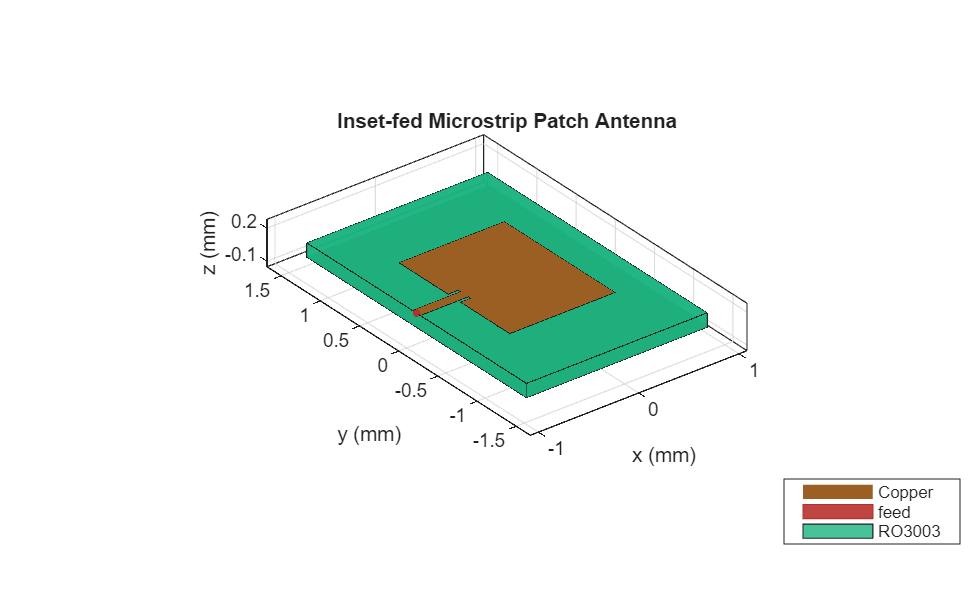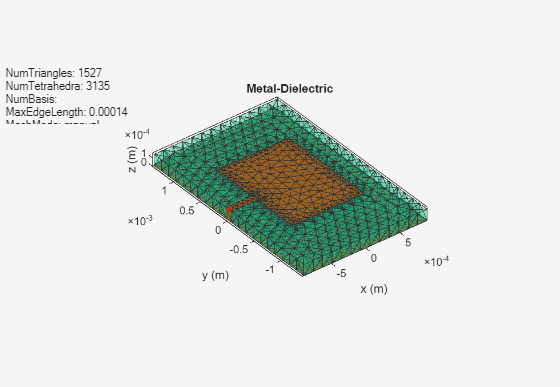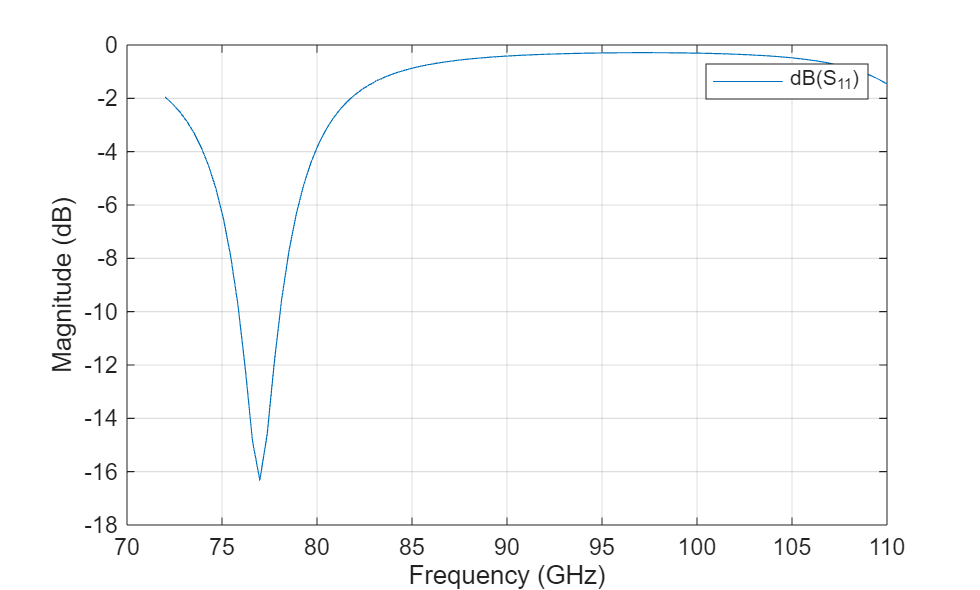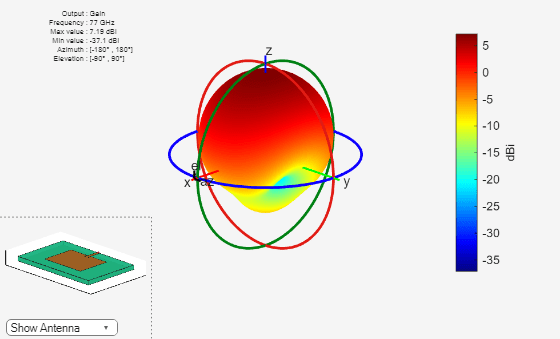This example shows how to create, model, and analyze an inset-fed patch microstrip antenna at high frequencies. As the frequency of operation increases to millimeter waves, the antenna sizes decrease and the antennas are fabricated on printed circuit boards (PCBs). Such printed antennas are of light weight, are inexpensive, easy to integrate, and are widely used as components in a radar. The antenna designed in this example operates at a frequency of 77 GHz and is used in an automotive radar receiver.

### Define Parameters

The length and the width of the patch are as provided in . The antenna is designed on a Rogers RO3003™ substrate, with the dielectric constant of `3`, loss tangent of `0.0013`, and `130` um thickness. A copper conductor of thickness `17` um is used as the patch.

```PL = 1049e-6; PW = 1412e-6; sub = dielectric(Name="RO3003", EpsilonR=3, LossTangent=0.0013, Thickness=130e-6); con = metal(Name="Copper", Conductivity=5.96e7, Thickness=17e-6);```

### Create Patch Microstrip Antenna

Create an inset-fed patch microstrip antenna using the `patchMicrostripInsetfed` object. The ground plane dimensions are `1800` um by `2800` um. The length and the width of the notch are specified as `100` um and `160` um, respectively. The strip line width is `100` um. The antenna feed is located at the end of the strip.

```ant = patchMicrostripInsetfed(Length=PL, Width=PW, Height=sub.Thickness,... Substrate=sub, Conductor=con, GroundPlaneLength=1800e-6,... GroundPlaneWidth=2800e-6, NotchLength=100e-6, NotchWidth=160e-6,... StripLineWidth=100e-6, FeedOffset=[-900e-6 0]); show(ant)```### Analyze Antenna

Use the `mesh` function to create and display the mesh structure of the patch microstrip antenna. Mesh the antenna with a maximum edge length of `140e-6` m.

`mesh(ant,MaxEdgeLength=140e-6) `

Use the `sparameters` function to plot the s-parameters of patch antenna over a frequency range of `72` -`110` GHz. The antenna resonates at 77 GHz, with an approximate bandwidth of 2.7 GHz

```sf = sparameters(ant,linspace(72e9,110e9,100)); figure; rfplot(sf)```Use the `pattern` function to plot the 3-D radiation pattern of the antenna at `77` GHz. The gain obtained from the antenna is over 7 dBi.

`pattern(ant,77e9)`Plot the copolarization plot in the H-plane for the antenna at `77` GHz in a rectangular coordinate system with normalization.

`pattern(ant,77e9,0,0:1:180,CoordinateSystem="rectangular",Normalize=true)`### Conclusion

The analysis results of the 77 GHz microstrip patch antenna show that it has good bandwidth and gain and is suitable for use in applications like automotive radar receivers.

### References

 Seyyedesfahlan, Mehdi and I. Tekin. “77 GHz PCB Patch Antenna.” URSI (International Union of Radio Science) Türkiye Ulusal Komitesi (2016).